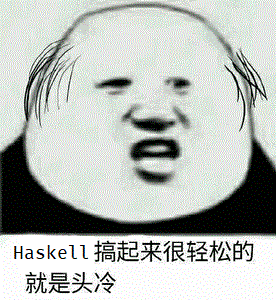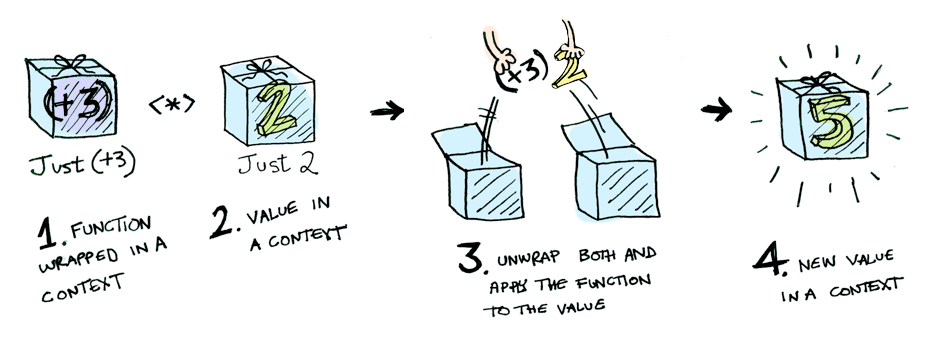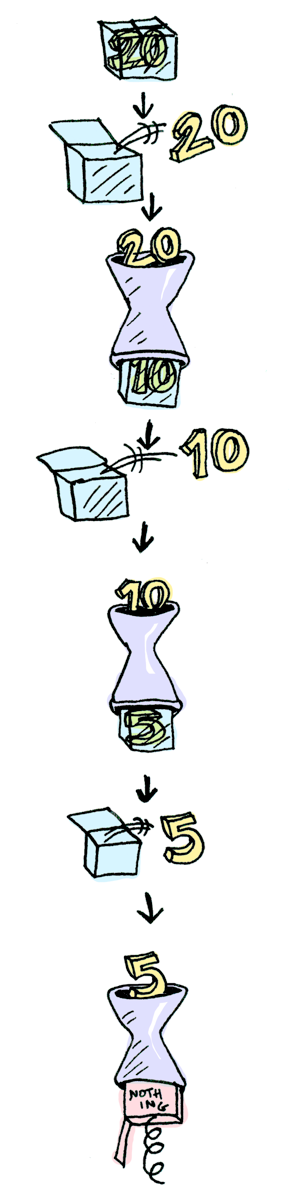book

flag
mode_edit

# 范畴论

## 范畴（Category）

• 一些东西O(Object)
• 两个东西间的关系，箭头~>(态射)

• 一定有一个叫 id 的箭头，也叫做 1
• 箭头可以组合

### 认识一些范畴

#### 示例图• 东西是类型
• 箭头是类型的变换，即 ->
• id 就是 id 函数的类型 a -> a
• compose 当然就是函数组合的类型

## 函子（Functor）

### 认识一些函子

#### 自函子# Applicative Functors

class (Functor f) => Applicative f where
pure :: a -> f a
(<*>) :: f (a -> b) -> f a -> f b<*>就比较蛇，这个东西是一个中缀的运算符，作用。。是把一个包在Functor里的函数应用到另一个Functor里。

ghci> pure (+42) <*> Just 1
Just 43
ghci> pure (++"qwq") <*> Just "233"
Just "233qwq"
ghci> pure (+) <*> Just 4 <*> Just 9
Just 13f <$> x = fmap f x  可以这样： ghci> (++) <$> Just "johntra" <*> Just "volta"
Just "johntravolta"


# Monoids

• 结合律：对任何在 $M$ 内的$a$、$b$、$c$ ， $(a\times b)\times c = a\times (b\times c)$ 。
• 单位元：存在一在 $M$ 内的元素$e$，使得任一于 $M$ 内的 $a$ 都会符合 $a\times e = e\times a = a$ 。

• 封闭性：对任何在 $M$ 内的 $a$，$b$ ， $a\times b$ 也会在 $M$ 内。

## 一些例子

### 妖精大战争

​ 琪露诺的斗志值。

​ 如果Miss一次将减少100%。

​ 在99%以下的状态时Miss，则游戏结束。

- 击伤敌人

增加100%等价于增加一个自机，最大1000%。

modify :: Int -> Int -> Int
modify before delta = before + delta
x -|> f = f x


100 -|> modify 10 -|> modify -100 -|> modify 20 -|> modify -100 -|> modify 20


modify :: Int -> Int -> Maybe Int
modify before delta
| before + delta > 0 = Just before + delta
| otherwise = Nothing


Maybe可以返回多种值，导致我们不得不去分开处理它们。

class Monad m where
return :: a -> m a
(>>=) :: m a -> (a -> m b) -> m b
(>>) :: m a -> m b -> m b

x >> y = x >>= \_ -> y
fail :: String -> m a
fail msg = error msg


>>=就比较皮，它读作“bind”，它接受一个装在盒子里的值，然后把值从盒子里拿出来，应用到一个函数上，返回装在盒子里的另一个值

instance Monad Maybe where
return x = Just x
Nothing >>= f = Nothing
Just x >>= f = f x
fail _ = Nothing


### 回到例子

return 100 >>= modify 10 >>= modify -100


### 另一个例子

half x = if even x
then Just (x div 2)
else Nothing


ghci> Just 20 >>= half >>= half >>= half
Nothing## do表示法

foo :: Maybe String
foo = do
x <- Just 3
y <- Just "!"
Just (show x ++ y)


<-实际上就是把盒子里的值取出来

main = do
qwq <- getline
print qwq